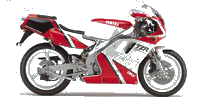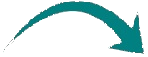TZR250 3MANew
Title
Go to contentSometimes things does not go as planned.
After a few update's on forums my dyno results where more positive than the average dyno result.
I could not find the problem until recalculated the drum it turns out that my software was not set correct so the measurements where incorrect.

Now with the new settings my measurements drops enormously

Sadly i don't have all the rough data anymore so can't set it all back. Only the data from the TZR125 I have stored.
From now
23-09-2017 all measurements will be done according the new setting

From the old ones seems to be you can only estimated it with roughly a 7HP lessHere we have the measured value's

D=390
h=300
metal=7900
shaft 125 x 40 (two times)

V=pi x r2 x h
Drum
V=3,14x(0,195x0,195)x0.300=0,03582x7900=282,978kg

Shaft (coming out off the drum)
V=3.14x(0,020x0,020)x0,125=0,000157x7900=1,2403kg (that two time) =2,4806kg

Starter plate
V=4,55kg

Total weight 2,4806+282,978+4,55=290,0086kg

I = m/2 x r2
I = 290,0086/2 x (0,195x0,195)=
5,51

Back to content